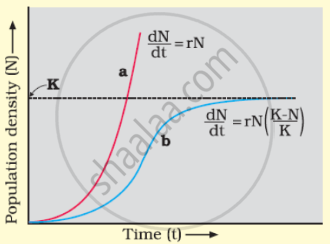# Answer the Following Question. Compare, Giving Reasons, the J-shaped and S-shaped Models of Population Growth of a Species. - Biology

Compare, giving reasons, the J-shaped and S-shaped models of population growth of a species.

#### Solution

There are 2 models of population growth:
(i) The exponential growth
(ii) Logistic growth

(i) Exponential growth:
This growth occurs where the resources (food + space) are unlimited. The equation can be represented as
(d"N")/(d"t") = ( b - d ) x N

Let ( b - d ) = r
(d"N")/(d"t") = rN or Nt = N0ert

N = population size
Nt = population density after time t.
N0 = population density at time zero
r = growth rate
b =birth rate
d = death rate
In this growth, when N in relation to time is plotted on the graph, the curve becomes J-shaped.

(ii) Logistics growth model:
This is a realistic approach as the resources become limited at a certain point of time.(a) J-shaped curve ⇒ exponential growth
(b) S-shaped curve ⇒ logistics growth

Every ecosystem has limited resources to support a particular maximum carrying capacity (K).
When N is plotted in relation to time t, a sigmoid-S-shaped curve is obtained & is also called as VERHULST-PEARL logistic growth.
The equation is:
(d"N")/(d"t") = "rN" (("K" - "N"))/("K")

N = population density at time t.
R = growth rate
K = carrying capacity.

Concept: Population Growth
Is there an error in this question or solution?